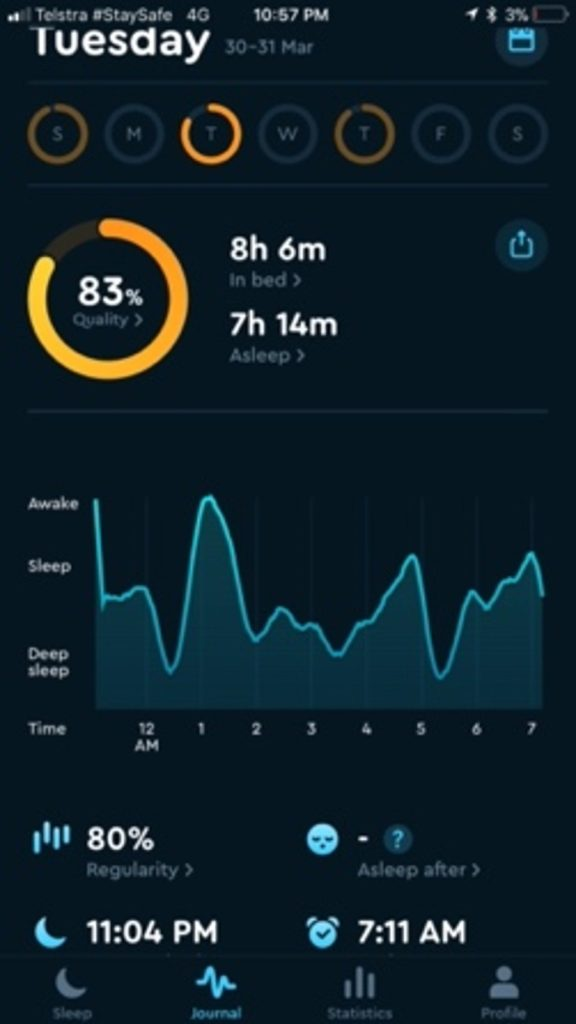# Problem of the Week## Quality Sleep

This reading from a sleep app tells the person that the quality of their sleep was 83% last night. Is this a percentage of the time that they were asleep while they were in bed? Could you tell by estimating?

If 83% was the time they were asleep as a percentage of the time they were in bed, it would be the fraction: 7hr 14min divided by 8hr 6min, expressed as a percentage (so multiplied by 100).

7hr 14min = 7 x 60 + 14 = 434min

8hr 6min = 8 x 60 + 6 = 486min

434/486 x 100 = 90% (to the nearest percent), which is not 83%.

A quicker way to work this out would be to estimate.

Rounding to the nearest hour:

7hr/8hr = 87.5%, which is not 83%.

To find 7 eighths as a percentage, you could think of it as 1 - one eighth.

A quarter is 25%. An eight is half of a quarter, so it is 12.5%.

100% - 12.5% = 87.5%.